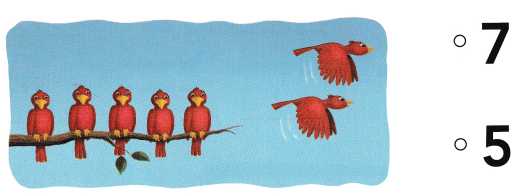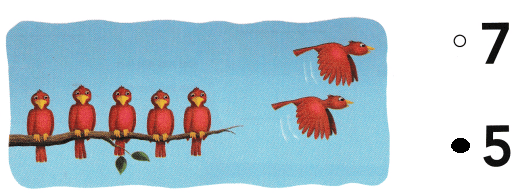# Texas Go Math Kindergarten Lesson 14.1 Answer Key Differences Within 7

Refer to our Texas Go Math Kindergarten Answer Key Pdf to score good marks in the exams. Test yourself by practicing the problems from Texas Go Math Kindergarten Lesson 14.1 Answer Key Differences Within 7.

## Texas Go Math Kindergarten Lesson 14.1 Answer Key Differences Within 7

Essential Question
How can you show differences within 7?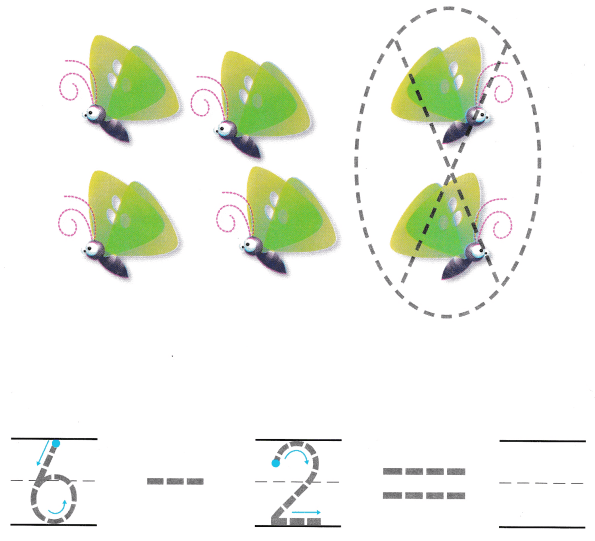Explanation:
There are six butterflies.
Two butterflies fly away.
6 – 2 = 4
so, 4 butter flies on the tree.

Directions
There are six butterflies. Two butterflies fly away. Trace the circle and X around those butterflies. How many butterflies are left? Trace and write to complete the subtraction sentence.

Share and Show

Question 1.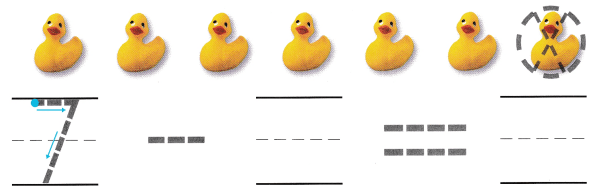Explanation:
There are 7 ducks
7 – 1 = 6
so, 6 ducks are left in the pond.

Question 2.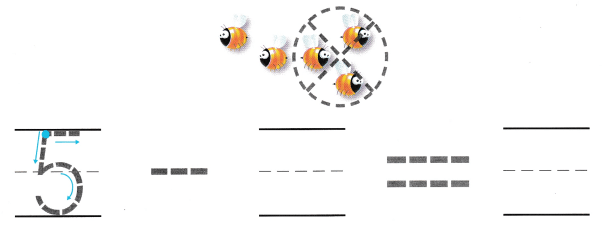Explanation:
There are 5 honey bees on the flower
3 went away from the flower
5 – 3 = 2
so, 2 honey bees are on the flower.

Question 3.Explanation:
There are 7 ants
3 ants went away
7 – 3 = 4
so, 4 ants are left.

Directions
1. Listen to the subtraction word problem. How many ducks swim away? Trace the circle and X. How many ducks are left? Trace and write to complete the subtraction sentence. 2-3. Listen to the subtraction word problem. Trace and write to complete the subtraction sentence.

Question 4.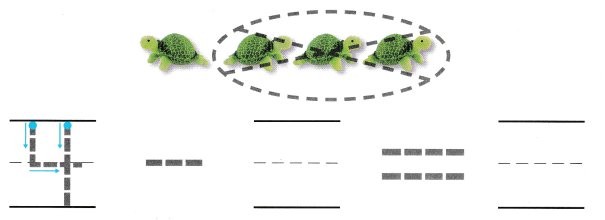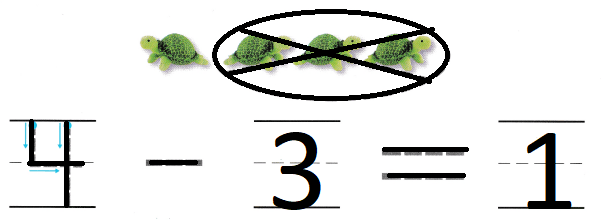Explanation:
There are 4 turtles
3 turtles run away
4 – 3 = 1
so, one turtle is left.

Question 5.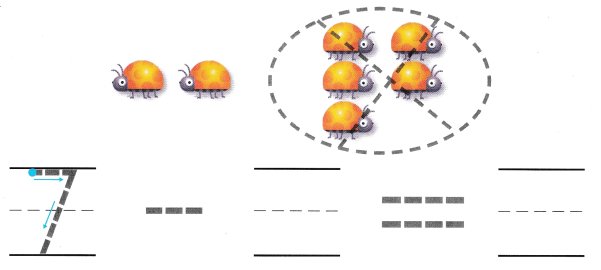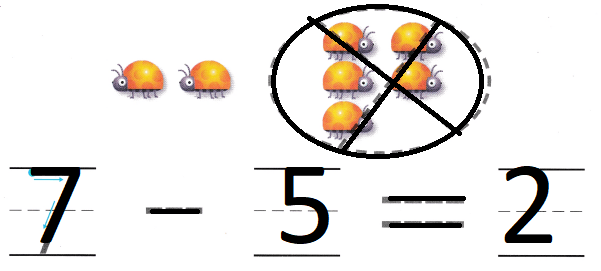Explanation:
5 crawl away
7 – 5 = 2
so, 2 lady bugs are left.

Question 6.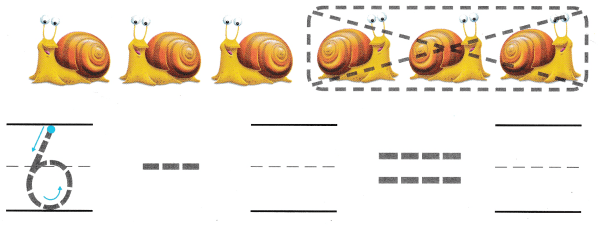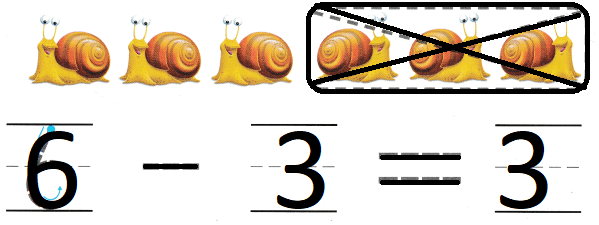Explanation:
There are 6 snails
3 snails swim away
6 – 3 = 3
so, 3 snails are left.

Directions
4-6. Listen to the subtraction word problem. Trace and write to complete the subtraction sentence.

Problem Solving

Question 7.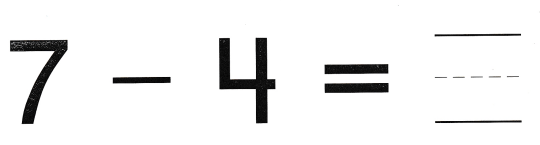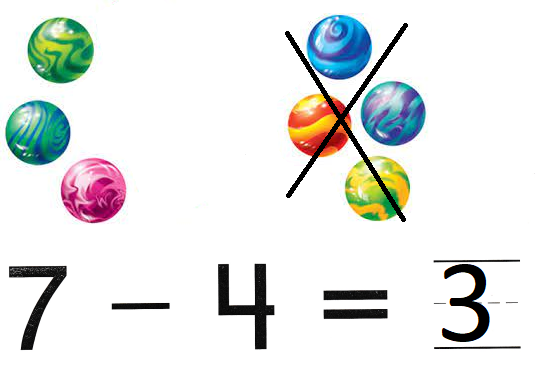Explanation:
There are 7 marbles with john
he gave 4 to his friend
7 – 4 = 3
so, john has 3 marbles left.

Question 8.Explanation:
There are six worms.
One worm crawls away.
6 – 1 = 5
so, 5 worms are left.

Directions
7. Complete the subtraction sentence. Draw to show what you know about this subtraction sentence. Tell a friend about your drawing. 8. Choose the correct answer. There are six worms. One worm crawls away. What is 6 – 1?

### Texas Go Math Kindergarten Lesson 14.1 Homework and Practice

Question 1.Explanation:
There are seven parrots.
Two parrots leave.
7 – 2 = 5
so, 5 parrots are left

Question 2.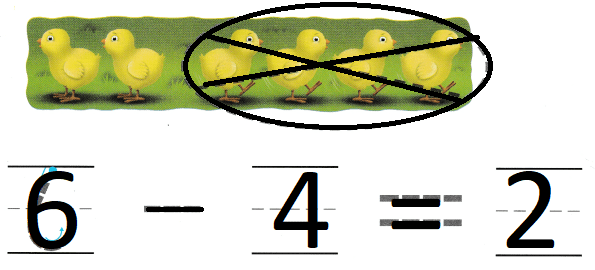Explanation:
There are six baby chicks.
Four chicks go to the barn.
6 – 4 = 2
so, 2 ducks are left.

Directions
Trace and write to complete the subtraction sentence. 1. There are seven parrots. Two parrots leave. 2. There are six baby chicks. Four chicks go to the barn.

Texas Test Prep

Lesson Check

Question 3.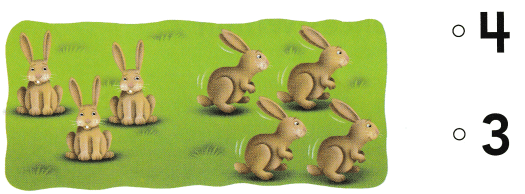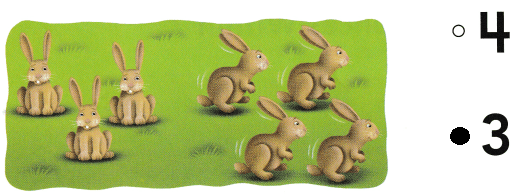Explanation:
There are seven rabbits.
Four rabbits hop away.
7 – 4 = 3
so, 3 rabbits are there

Question 4.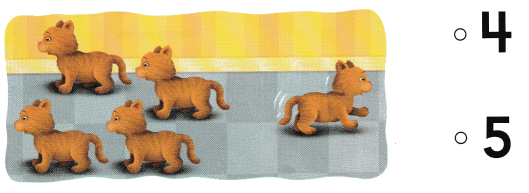Explanation:
There are five kittens.
One kitten leaves.
5 – 4 = 1
so, 1 kitten left.

Question 5.